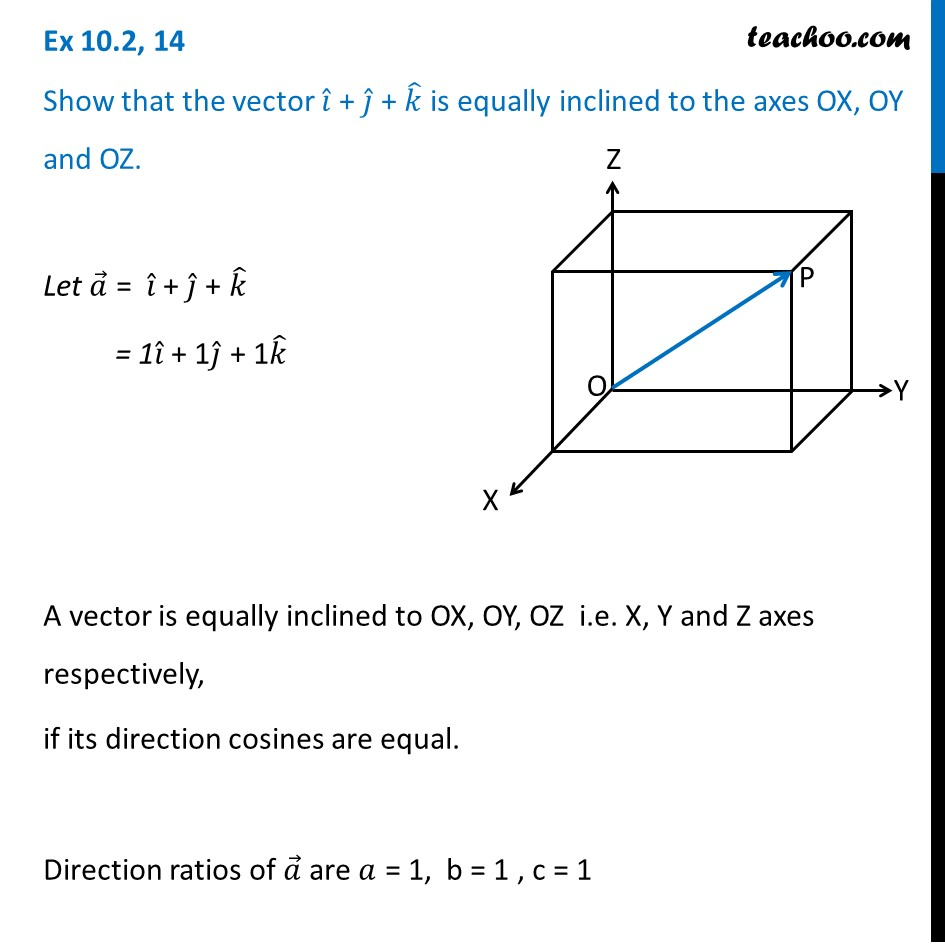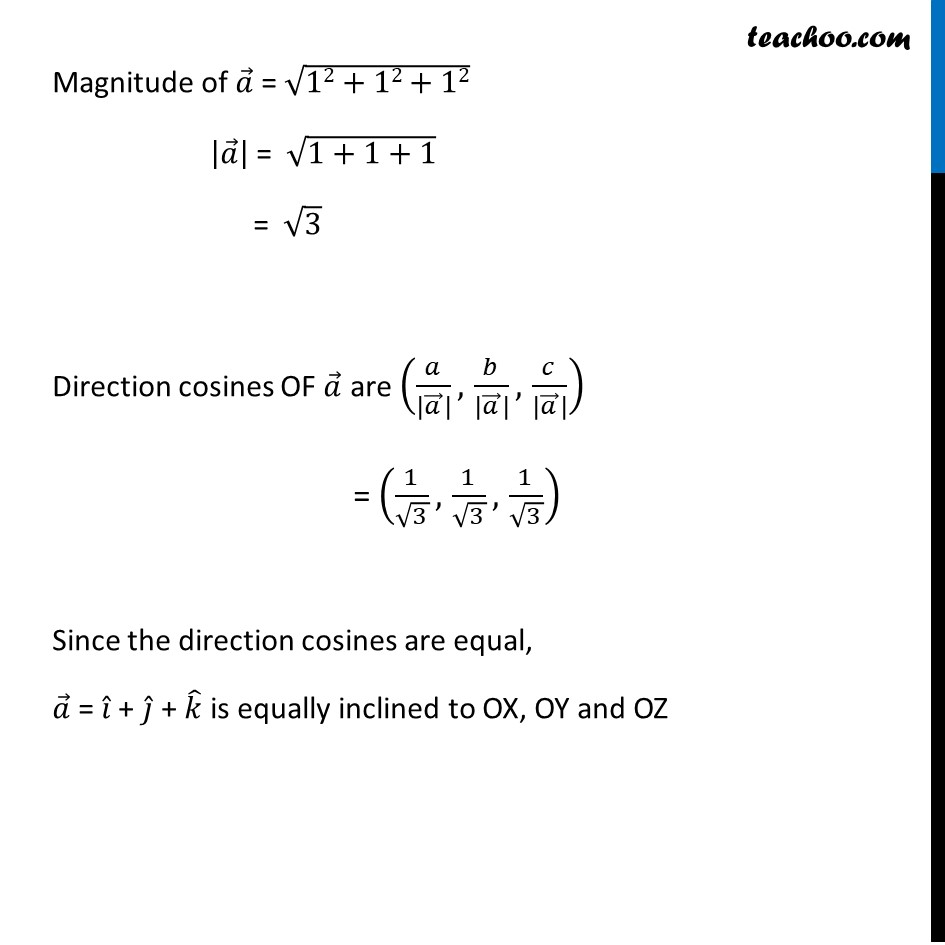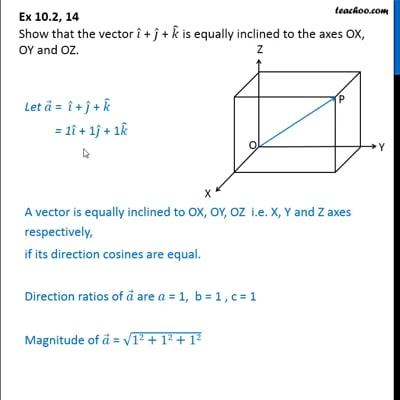Ex 10.2

Chapter 10 Class 12 Vector Algebra
Serial order wiseThis video is only available for Teachoo black users

Introducing your new favourite teacher - Teachoo Black, at only ₹83 per month

### Transcript

Ex 10.2, 14 Show that the vector 𝑖 ̂ + 𝑗 ̂ + 𝑘 ̂ is equally inclined to the axes OX, OY and OZ. Let 𝑎 ⃗ = 𝑖 ̂ + 𝑗 ̂ + 𝑘 ̂ = 1𝑖 ̂ + 1𝑗 ̂ + 1𝑘 ̂ A vector is equally inclined to OX, OY, OZ i.e. X, Y and Z axes respectively, if its direction cosines are equal. Direction ratios of 𝑎 ⃗ are 𝑎 = 1, b = 1 , c = 1 Magnitude of 𝑎 ⃗ = √(12+12+12) |𝑎 ⃗ | = √(1+1+1) = √3 Direction cosines OF 𝑎 ⃗ are (𝑎/|𝑎 ⃗ | ,𝑏/|𝑎 ⃗ | ,𝑐/|𝑎 ⃗ | ) = (1/√3,1/√3,1/√3) Since the direction cosines are equal, 𝑎 ⃗ = 𝑖 ̂ + 𝑗 ̂ + 𝑘 ̂ is equally inclined to OX, OY and OZ